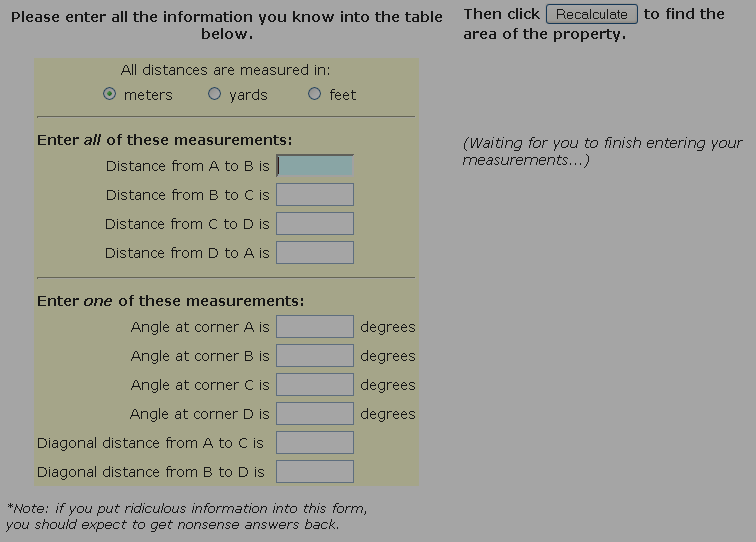SEARCH HOMEMath Central Quandaries & QueriesQuestion from many people: I have a real estate property and the lot size is something I need to find out. I know the lengths of the four sides, but it isn't a rectangle, it is an odd shape. How do I find the acreage?We often get questions about the area of parcels of land. We've created a tool (below) that should help you if your property has four sides you can measure. In addition to the four sides, we'll need to know either a diagonal or the degrees of one of the angles. Note that this tool assumes the shape is convex, not concave.

Start by choosing one corner of your property to be corner A. Then label the other three corners clockwise as B, C and D.

Please enter all your information into the form below. Then click to find the area of the property.
 All distances are measured in: meters      yards       feet Enter all of these measurements: Distance from A to B is Distance from B to C is Distance from C to D is Distance from D to A is Enter one of these measurements: Angle at corner A is degrees Angle at corner B is degrees Angle at corner C is degrees Angle at corner D is degrees Diagonal distance from A to C is Diagonal distance from B to D is

*Note: if you put ridiculous information into this form,
you should expect to get nonsense answers back.

(Waiting for you to finish entering your measurements...)

Not enough information.

You haven't entered enough information to calculate the area. Many people think that just the lengths of the sides matter, but shape matters too so we need at least one angle or diagonal length to define the shape.

Calculating...

Sorry. This tool requires Javascript which you have turned off in your browser.
Enable javascript and reload this page to use the lot size calculator.Math Central is supported by the University of Regina and The Pacific Institute for the Mathematical Sciences.